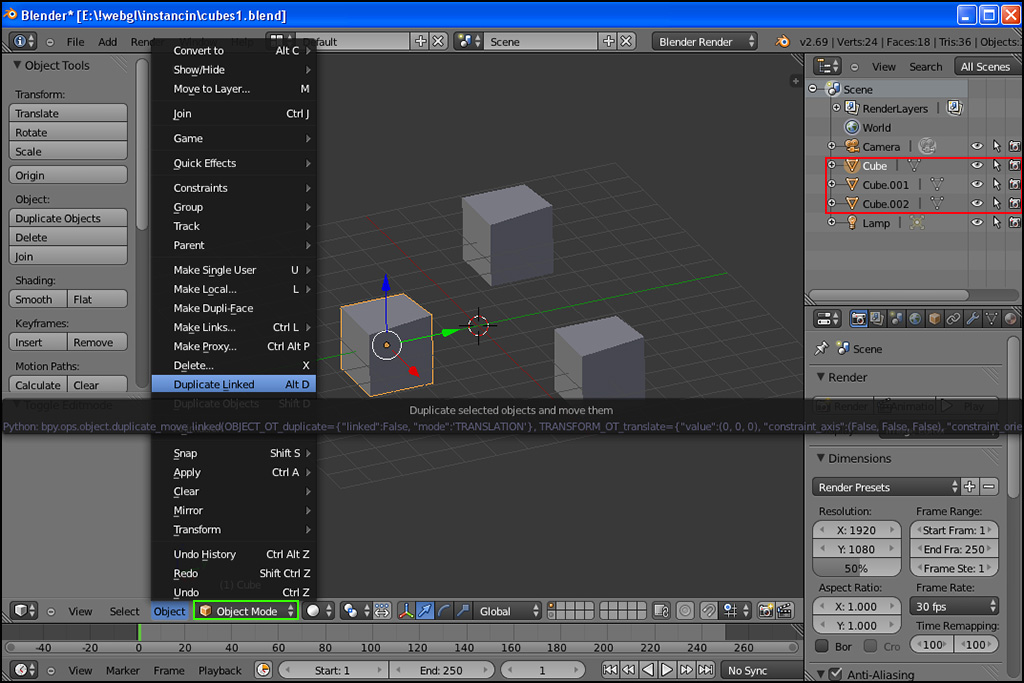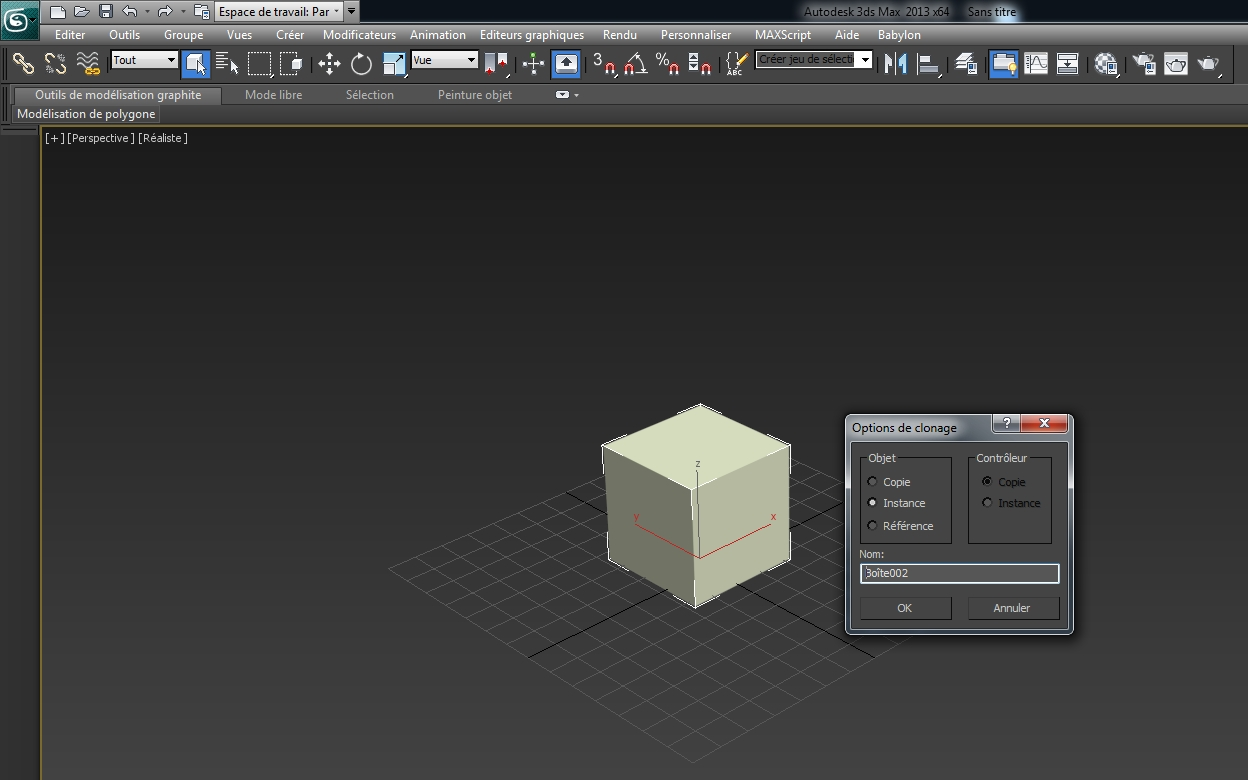# How to use Instances

Instances are an excellent way to use hardware accelerated rendering to draw a huge number of identical meshes (let's imagine a forest or an army).

Instances are built from a mesh with the following code:

``````// In this case we're loading our mesh from an external source.
BABYLON.SceneLoader.ImportMesh("", "//www.babylonjs.com/assets/Tree/", "tree.babylon", scene, function (newMeshes) {
var mesh = newMeshes;
// Make the "root" mesh not visible. The instanced versions of it that we
// create below will be visible.
mesh.isVisible = false;
for (var index = 0; index < 100; index++) {
var newInstance = mesh.createInstance("i" + index);
// Here you could change the properties of your individual instance,
// for example to form a diagonal line of instances:
//  newInstance.position.x = index;
//  newInstance.position.z = index;
// See below for more details on what can be changed.
}
});
``````

A mesh can have as many instances as you want.

Each instance has the same material as the root mesh. They can vary on the following properties:

• `position`
• `rotation`
• `rotationQuaternion`
• `setPivotMatrix`
• `scaling`

Note: related are thin instances, if you want yet more performances but with less control on each instance. See the dedicated page for further information.

# Instancing a glTF object

When you instanciate a glTF object, you need to make sure that the new instance will be under the same parent or you need to remove the parent from the source object.

This is because every gltf file comes from a right handed world. To get it into Babylon.js left handed world, we are adding an arbitrary parent that is adding a negative scale on z.

So when instancing a glTF object you have to (either):

• Call `source.setParent(null)`
• Or call `newInstance.setParent(source.parent)`

# Custom buffers

You also have the opportunity to specify per instance values for any attribute. For instance (no pun intended), if you want to have a specific color per instance, you only need to provide a vertex buffer flagged as "instanceable" and fill it with a color per instance:

``````let instanceCount = 1000;
let colorData = new Float32Array(4 * instanceCount);

for (var index = 0; index < instanceCount; index++) {
colorData[index * 4] = Math.random();
colorData[index * 4 + 1] = Math.random();
colorData[index * 4 + 2] = Math.random();
colorData[index * 4 + 3] = 1.0;
}

var buffer = new BABYLON.VertexBuffer(engine, colorData, BABYLON.VertexBuffer.ColorKind, false, false, 4, true);
box.setVerticesBuffer(buffer);
``````

The last parameter of the VertexBuffer constructor is the one to set to true to flag it as instanceable.

The other way is to register a custom buffer with `registerInstancedBuffer`:

``````mesh.registerInstancedBuffer("color", 4); // 4 is the stride size eg. 4 floats here
``````

Using this API, you can specify a new buffer that will be instancied. This means that each instance will have its own value. You can specify this value on the root mesh and on every single instance:

``````box.instancedBuffers.color = new BABYLON.Color4(Math.random(), Math.random(), Math.random(), 1);
let instance = box.createInstance("box1");
instance.instancedBuffers.color = new BABYLON.Color4(Math.random(), Math.random(), Math.random(), 1);
``````

The system will take care of updating the internal vertex buffer.

You can decide to control the world matrix instanced buffer the same way you control the custom buffers.

To do so, just run the following code:

``````mesh.manualUpdateOfWorldMatrixInstancedBuffer = true;
``````

When this mode is activated, you can update the world matrix instanced buffer with this code:

``````mesh.worldMatrixInstancedBuffer.set(mat, offset); // mat is the matrix you want to store at the given offset
offset += 16; (a matrix is composed of 16 floats
``````

It is recommended to freeze the active meshes when controling the world matrix instanced buffer to avoid having a discrepancy between the values you store and the number of active instances:

``````scene.freezeActiveMeshes(true);
``````

You can find a complete example here: https://www.babylonjs-playground.com/#HJGC2G -

# Support

Instances are supported for collisions, picking, rendering and shadows. Even if the current hardware does not support hardware accelerated instances, babylon.js will be able to optimize rendering to take instances into account.

# Using 3D modeler to create instances

## Blender

Using Blender, you can create instances of a mesh by just creating a linked object:## 3DS Max

Using 3DS Max, you can create instances of a mesh by just creating a clone instance object with clic right on the object:# Limitations

• You can use instances with LOD but one limitation will apply in this case: You will have to hide the root objects. Here is an example where LODs reuse instances: https://www.babylonjs-playground.com/#0720FC#10 -

• If you want to create an instance from a cloned mesh, you have to first make sure that you call clonedMesh.makeGeometryUnique().

• Instances with a world matrix where determinant is different than root mesh world matrix will be rendered separately (like a regular mesh). This mostly happens when the sign of the scaling vector is different between an instance and the root mesh.